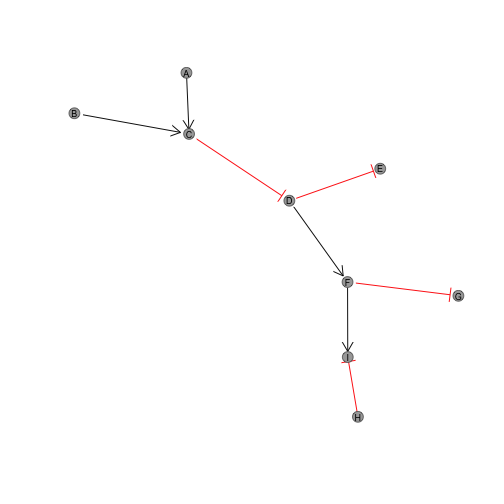## Install

``````library("igraph")
library("graphsim")
``````

##Set up simulated graph

``````graph_edges <- rbind(c("A", "C"), c("B", "C"), c("C", "D"), c("D", "E"), c("D", "F"), c("F", "G"), c("F", "I"), c("H", "I"))
graph <- graph.edgelist(graph_edges, directed = T)
``````

##Plotting

`plot_directed` with default settings uses the `layout.fruchterman.reingold` as does the default `igraph::graphsim`.

``````plot(graph)
``````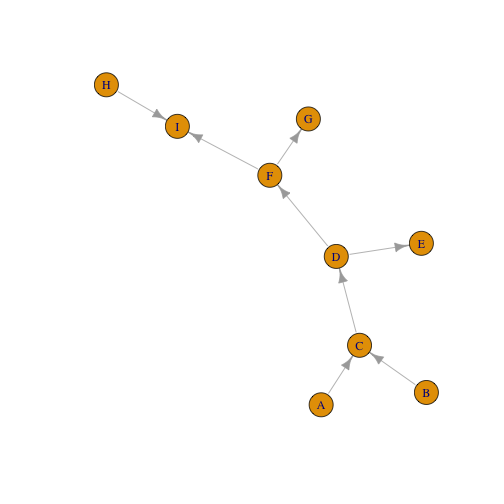``````plot_directed(graph)
``````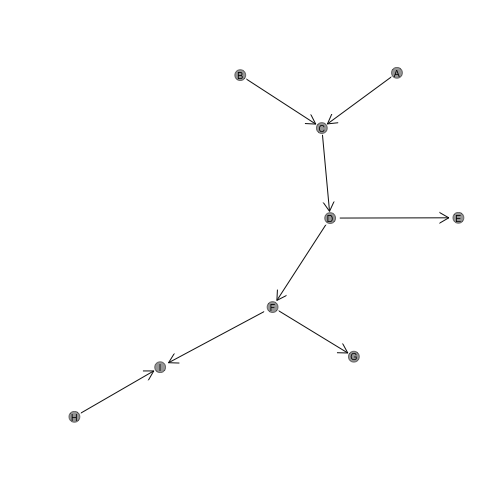`plot_directed` supports customised layouts and colours:

``````plot_directed(graph, layout = layout.kamada.kawai)
``````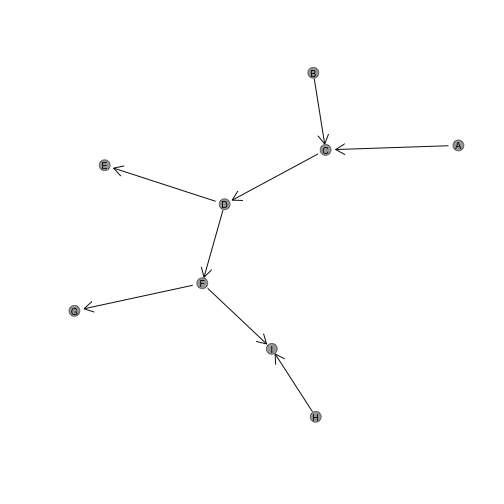``````plot_directed(graph, fill.node = "lightblue", border.node = "royalblue")
``````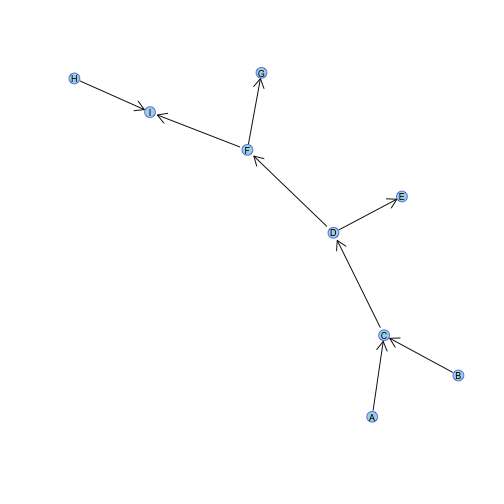Colours may also be entered as a vector for each node in `V(graph)`:

``````names(V(graph))
``````
``````##  "A" "C" "B" "D" "E" "F" "G" "I" "H"
``````
``````colour_vector <- ifelse(names(V(graph)) %in% c("A", "D", "I"), 1, 2)
plot_directed(graph, fill.node = c("lightblue", "grey")[colour_vector], border.node = c("royalblue", "black")[colour_vector])
``````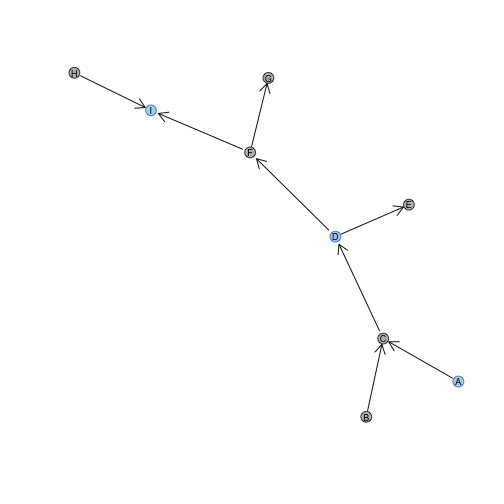##Arrow customisation

The `state` parameter controls whether the links are “activating” or “inhibiting”. These may be applied globally as a character or numeric:

• “activating”
• 1
• 0

• “inhibiting”
• -1
• 2
``````plot_directed(graph, state = "activating")
``````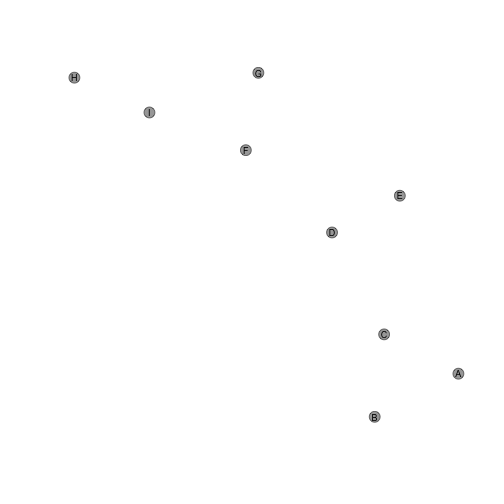``````plot_directed(graph, state = "inhibiting")
``````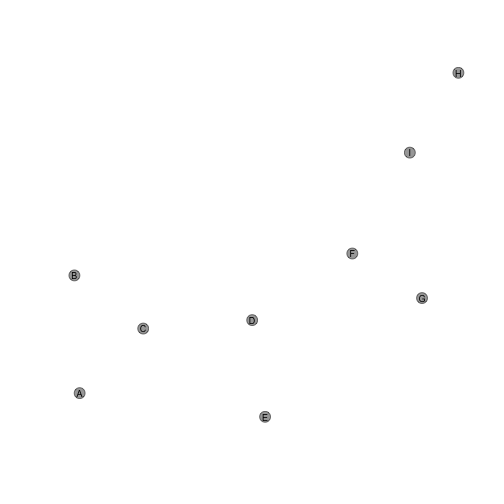``````plot_directed(graph, state = 1)
``````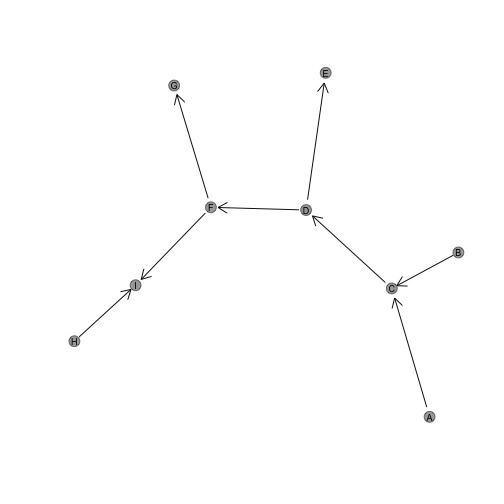``````plot_directed(graph, state = -1)
``````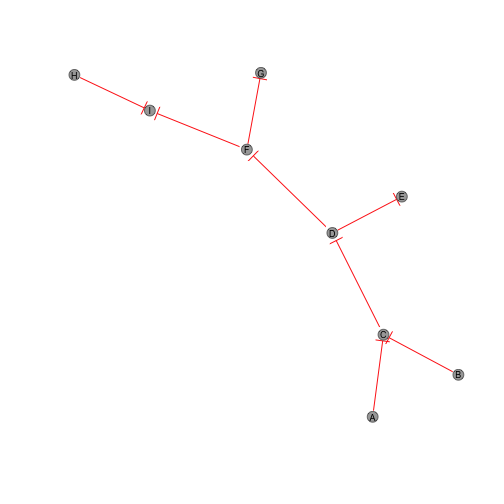``````plot_directed(graph, state = 0)
``````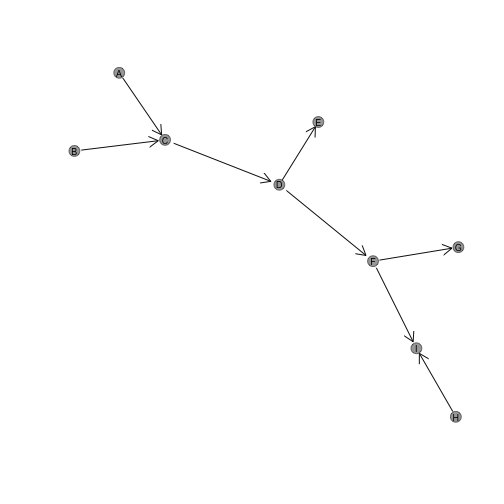``````plot_directed(graph, state = 2)
``````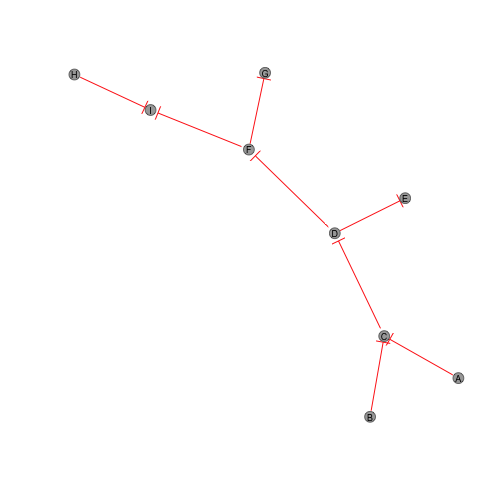#Vectorisation

The state parameter may also apply as a vector to each edge in E(graph) respectively.

``````E(graph)
``````
``````## + 8/8 edges from abc5aa9 (vertex names):
##  A->C B->C C->D D->E D->F F->G F->I H->I
``````
``````plot_directed(graph, state = c(1, 1, -1, -1, 1, -1, 1, -1))
``````